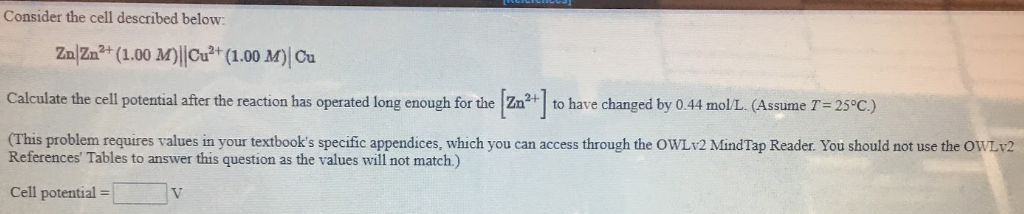# Consider the cell described below: Zn|Zn^2+ (1.00 M)||Cu^2+(1.00 M)| Cu Calculate the cell potential after the reaction has operated long enough for the [Zn^2+] to have changed by 0.44 mol/L. (Assume T = 25 °C.) (This problem requires values in your textbook's specific appendices, which you can access through the OWLv2 Mindtap Reader. You should not use the OWLv2 References' Tables to answer this question as the values will not match.)